# Correlation Of Mass Transfer Coefficients For Single Cylinder Chemical Engineering Notes | EduRev

## Chemical Engineering : Correlation Of Mass Transfer Coefficients For Single Cylinder Chemical Engineering Notes | EduRev

The document Correlation Of Mass Transfer Coefficients For Single Cylinder Chemical Engineering Notes | EduRev is a part of the Chemical Engineering Course Mass Transfer.
All you need of Chemical Engineering at this link: Chemical Engineering

3.4.3 Correlation of mass transfer coefficients for single cylinder
Bedingfield and Drew (1950) studied the sublimation from a solid cylinder into air which is flowing normal to its axis. They developed a correlation for the mass transfer coefficient from their experimental data which can be represented as: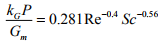(3.42)
In this case the Reynolds number is defined based on the diameter of the cylinder. Gm is the molar mass velocity of the gas and P is the total pressure. The correlation is applicable in the range of 400 < Re < 25000 and 0.6 < Sc < 2.6.

3.4.4 Correlation of mass transfer coefficients in circular pipes
In a wetted wall towers as shown in Figure 3.1, the mass transfer from the thin liquid film in the tube wall from the moving fluid has been studied extensively. In the tower a volatile pure liquid flows down inside the surface of the tube wall where a gas is allowed to pass through the central core. Here the evaporation of the liquid into the moving gas stream through the gas liquid surface is referred as mass transfer from liquid to gas Gilliland and Sherwood (1934) developed the following correlation from the experimental data obtained by using different liquid and air as gas in the range of 2.0×10< Re < 3.50×103 and 0.6 < Sc < 2.5.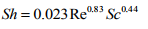(3.43)
where the physical properties of the gas are evaluated at the bulk conditions of the moving gas. Sherwood number and Reynolds number are based on tower diameter. Linton and Sherwood (1950) studied the mass transfer by extending the Schmidt number. They developed a correlation with the extended data set and the data set of Gilliland and Sherwood which can be represented as: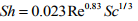(3.44)
In the range of 0.4×104 < Re < 7.0×104 and 0.6 < Sc < 3.0×103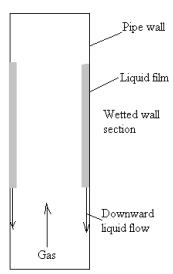Figure 3.1: Wetted-wall tower

3.4.5 Correlation of mass transfer coefficients in packed and fluidized beds
Based on advantages of increase surface area available for mass and heat transfer for a given volume compared to an empty vessel, packed and fluidized beds are commonly used in industrial mass transfer operations. For packed and fluidized beds, the area of mass transfer is generally expressed in terms of specific interfacial area which is defined as the area per unit volume of packed bed. It can e expressed as: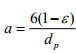(3.45)
Where ε is the porosity or void fraction and dp is the particle diameter. Various investigations have been carried out for estimating the mass transfer coefficients in packed beds and developed correlations for mass transfer coefficient from their experimental results. For both gas and liquid packed and fluidized bed of spherical particle, Gupta and Thodos (1962) developed a correlation which can be expressed as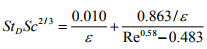(3.46)
The correlation is valid only in the range of 1 < Re < 2100. Sherwood et al. (1975) developed the following correlation for gases in the range of 10 < Re < 2500.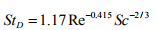(3.47)
where Re = (dpGy)/µ. Gy is the mass velocity of gas based on total cross sectional area of the tower and dp is the diameter of a sphere with the same surface area per unit volume as the particle. Stanton number (StD) for mass transfer is defined as (Sh/(Re.Sc)).

3.4.6 Mass transfer coefficient in hollow-fiber membrane module
Hollow-fiber membrane modules are extensively used in various membrane separation processes including gas separation, reverse osmosis, filtration and dialysis. In this module a bundle of randomly packed fibers are enclosed in a case to contact two process streams. As per Bao et al. (1999), a typical hollowfiber membrane module is shown in Figure 3.2. When fluids flow through the shell and lumen as shown in Figure, mass is transferred from one stream to other stream through the fiber wall. The mass transfer depends on the nature of the membrane. There are numerous correlations in literature for shell side mass transfer coefficients in this module of membrane.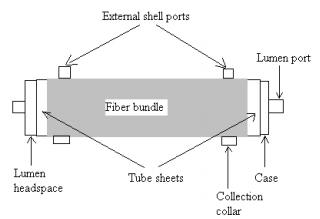Figure 3.2: Typical hollow fiber membrane

Costello et al. (1993) developed a correlation for mass transfer coefficient for liquid flowing through the shell parallel to the fiber which can be represented as: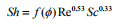(3.48)
where the packing fraction,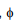refers to the fraction of the cross sectional area that is occupied by the fibers.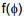is the function of fiber packing fraction which is written as: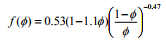(3.49)
The Sherwood number and Reynolds numbers are defined as 2RkL/DAB and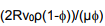respectively. R is the fiber radius and v0 is the superficial liquid velocity. The above correlation is valid in the range of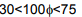and 20In case of flow through the lumen and constant wall concentration which is more common in application, Cussler (1997) made a correlation which is given by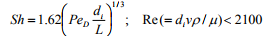(3.50)
where Sh = dikL/DAB is the Sherwood number for module length L. di is the fiber inside diameter. The correlations are also given in tabular form in Table 3.2.
Table 3.2: Correlations for mass transfer coefficient for different cases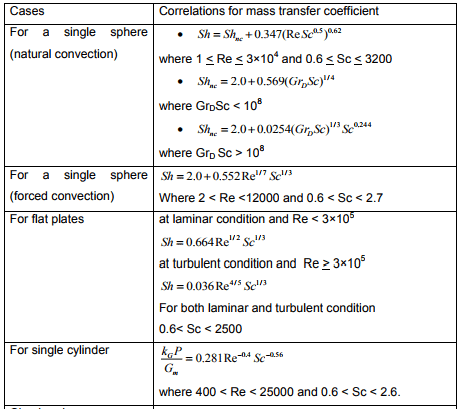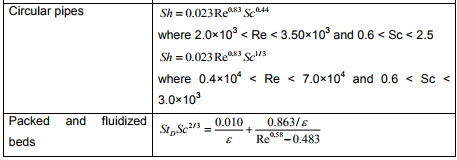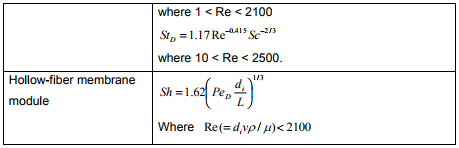Offer running on EduRev: Apply code STAYHOME200 to get INR 200 off on our premium plan EduRev Infinity!

## Mass Transfer

29 videos|46 docs|44 tests

,

,

,

,

,

,

,

,

,

,

,

,

,

,

,

,

,

,

,

,

,

;